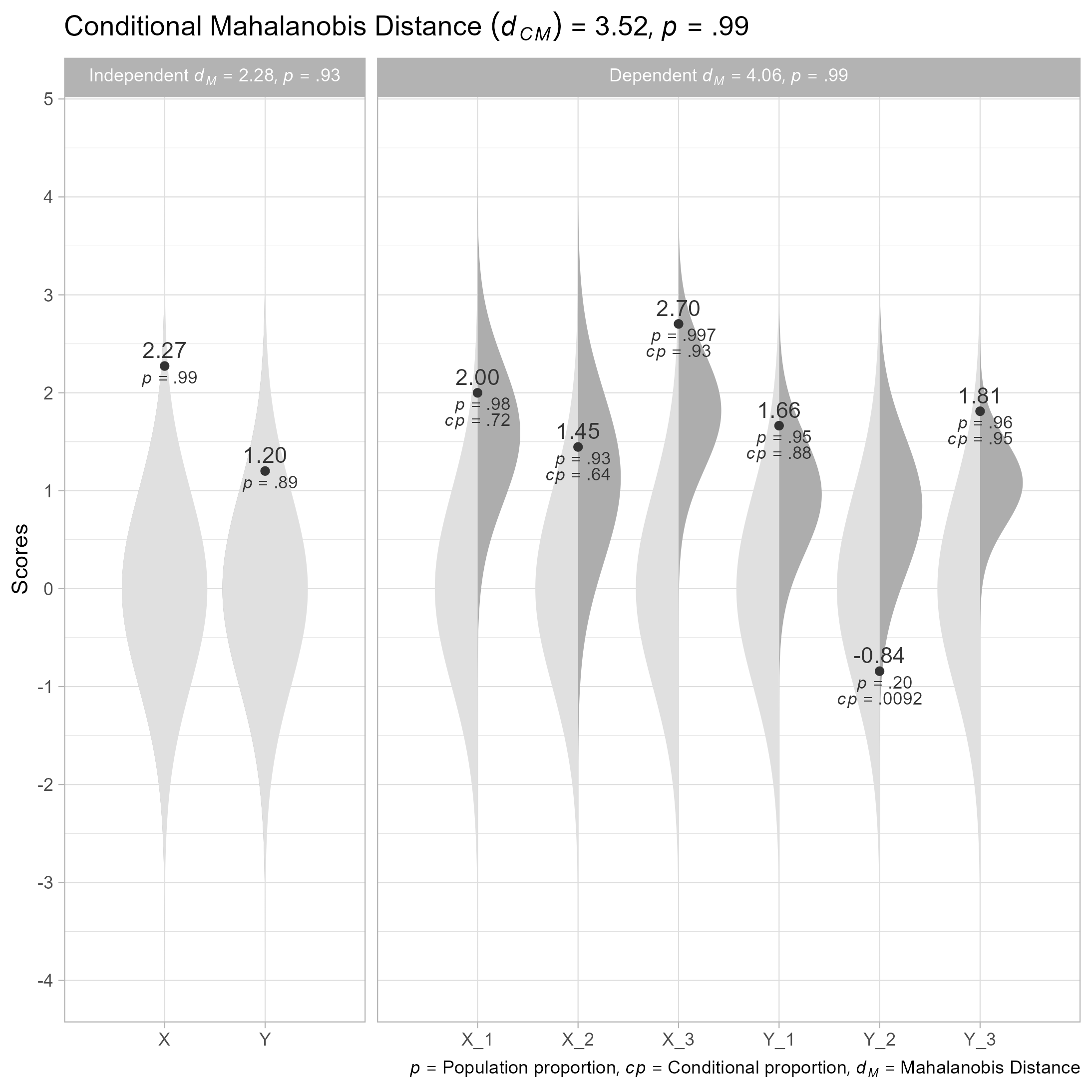# unusualprofileThe goal of unusualprofile is to calculate conditional Mahalanobis distances and related statistics. Such statistics can help find cases that are unusual, even after controlling for specified predictors.

# Installation

You can install the development version from GitHub with:

``````# install.packages("remotes")
remotes::install_github("wjschne/unusualprofile")``````

# Example

To use the unusualprofile package, one needs to know the correlations, means, and standard deviations among a set of continuous variables and at least one row of data from that set of variables.

Suppose we have set of variables that have the following relationships:

First, we load the unusualprofile package.

``library(unusualprofile)``

Included with the unusualprofile package, the `d_example` data set has a single row of data generated from the path diagram depicted above.

``````#>        X_1     X_2      X_3      Y_1        Y_2      Y_3        X        Y
#> 1 1.999498 1.44683 2.703249 1.664106 -0.8427126 1.811528 2.273441 1.201208``````

Also included with the unusualprofile package is the path diagram’s model-implied correlation matrix:

``````R_example
#>       X_1  X_2   X_3   Y_1   Y_2   Y_3    X    Y
#> X_1 1.000 0.35 0.560 0.336 0.294 0.378 0.70 0.42
#> X_2 0.350 1.00 0.400 0.240 0.210 0.270 0.50 0.30
#> X_3 0.560 0.40 1.000 0.384 0.336 0.432 0.80 0.48
#> Y_1 0.336 0.24 0.384 1.000 0.560 0.720 0.48 0.80
#> Y_2 0.294 0.21 0.336 0.560 1.000 0.630 0.42 0.70
#> Y_3 0.378 0.27 0.432 0.720 0.630 1.000 0.54 0.90
#> X   0.700 0.50 0.800 0.480 0.420 0.540 1.00 0.60
#> Y   0.420 0.30 0.480 0.800 0.700 0.900 0.60 1.00``````

## Using the `cond_maha` function

We can specify the correlations (`R`), means (`mu`), standard deviations (`sigma`). independent variables (`v_ind`), and dependent variables (`v_dep`). In this case, the independent variables are composite scores summarizing the dependent variables.

``````# Conditional Mahalanobis distance
cm <- cond_maha(data = d_example,
R = R_example,
mu = 0,
sigma = 1,
v_ind_composites = c("X", "Y"),
v_dep = c("X_1", "X_2", "X_3",
"Y_1", "Y_2", "Y_3"))

cm
#> Conditional Mahalanobis Distance = 3.5167, df = 4, p = 0.9852

# Plot
plot(cm)``````